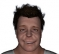lol

## Re: Spectrogram and room correction question.

Cool, will check it out.Thanks..

## Re: Spectrogram and room correction question.

Ah Ha!? I was thinking spectrum analyzer and RTA were the same thing.So an RTA is a spectrum analyzer that reverses the pink noise falloff of 10db? (basically undoes...

## Re: Need help - Pink noise analyzer

ThanksNow how do I give beers in here. :)

## Re: Need help - Pink noise analyzer

From this post here it says "each element shows the relative amplitude (0..1) of a the frequency equal to **N 24000 / Q* Hertz"It looks like the values represented...

...

## Spectrogram and room correction question.

When setting up a room for a flat frequency response using pink noise does the spectrum analyzer need to be setup a certain way so that it gives the proper flat...

## Re: Need help - Pink noise analyzer

Think I got it now. Using your answer and Oliviert's I think I might have it. float energy = Mathf.Pow(Mathf.Abs(fftResultBins[i] - (1f / currentFrequency)), 2);               ...

## Re: Need help - Pink noise analyzer

float energy = Mathf.Pow(fftResultBins[i], 2);                b[binIndex] += energy; Without dividing by the number...

## Re: Need help - Pink noise analyzer

Here are the 2 noise types zoomed in with the mean. I see what you mean.Pink is not flat but white is. I will try the other next.white noisePink noise

## Re: Need help - Pink noise analyzer

That is getting me a flat response! Thank you...I switched the code to this: (and divide the bin once full by the number of values I put in the bin.float energy...

## Re: Need help - Pink noise analyzer

Does it not look right because it is linear? Here it is in log. Green is fft results and blue is 1/f. (The results are better but as seen above, the graph is still...

## Re: Need help - Pink noise analyzer

Thanks for the quick reply! Did I understand correctly in the code below? I subtract 1/currentFrequency from each frequency that I am adding to the bins? I have...

## Need help - Pink noise analyzer

Hello, I am making a 31 band pink noise analyzer.  I send the pink noise file through a windowed FFT. I then take the frequency data and add the values in each...

Use this form to contact FreeGameDev

Before you can contact a member of the *Related Sites:

• You must be logged in (register here)
• You must confirm you email address